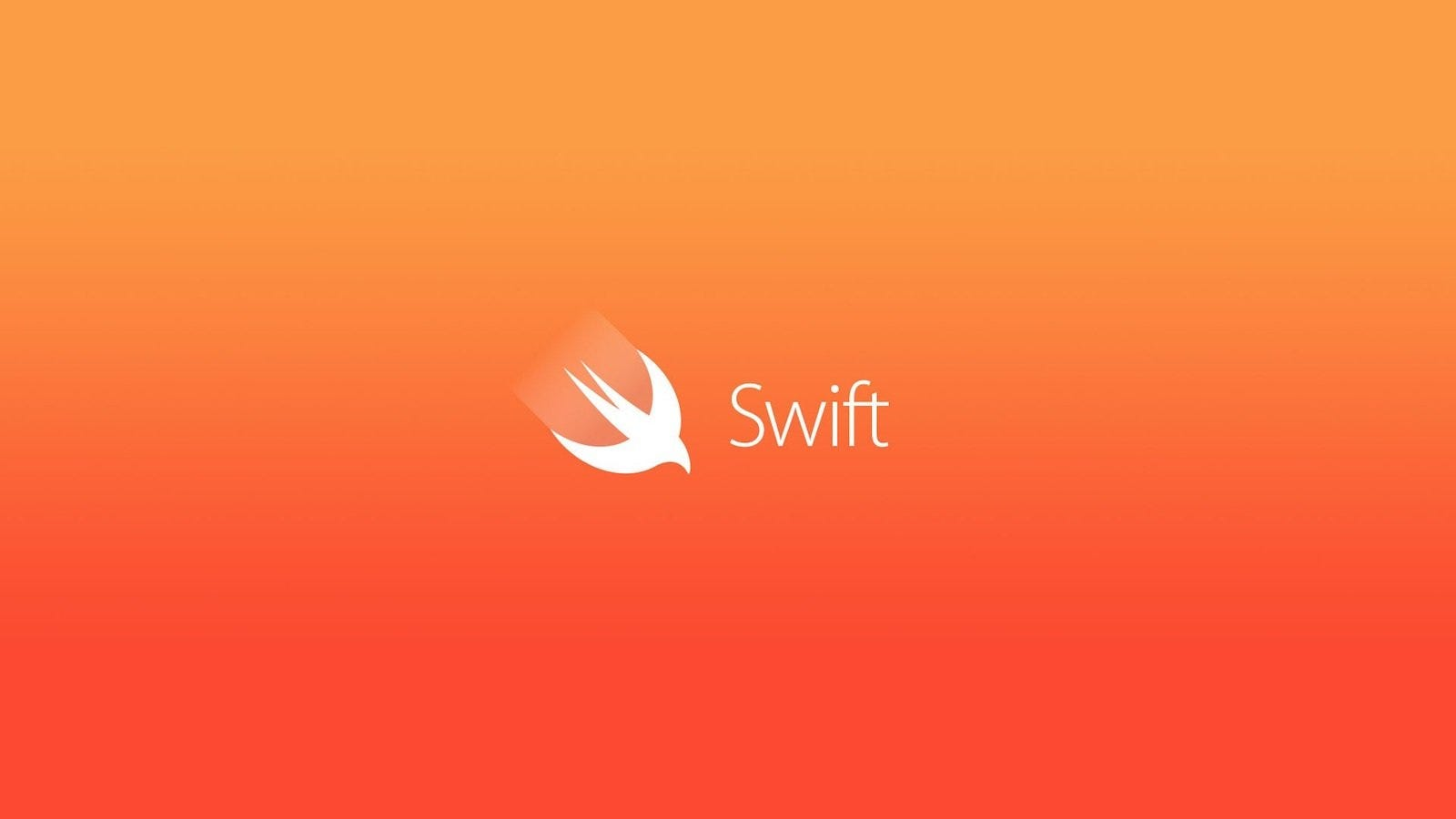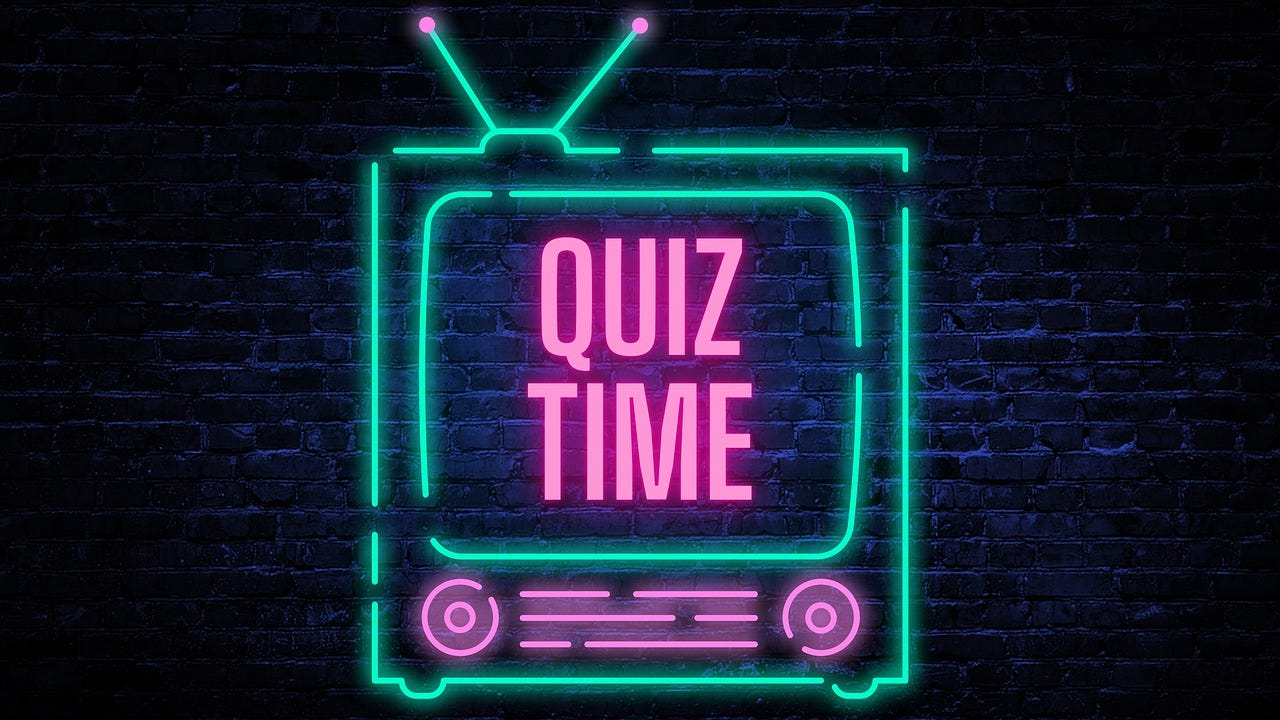# Swift Quiz #04: Swift Strings and Characters

Topics: String literals, Characters, and String manipulations

## Quick Refresher:1. String literals: String can be created by enclosing a sequence of characters within double quotation marks. For example:

``````    let greeting = "Hello, world!"
``````

2. Multiline string literals: Multiline strings can be created by enclosing the characters within three double quotation marks. Line breaks and indentation are preserved in multiline strings. For example:

``````    let multilineString = """
This is a
multiline
string.
"""
``````

3. Special characters: String literals can include special characters such as newline (\n), tab (\t), and Unicode scalar values . For example:

``````    let specialString = "Special characters: \n\t\\u{2665}"
``````

4. String interpolation: You can include variables, constants, expressions, and literals within a string using string interpolation. It’s denoted by the backslash followed by parentheses within a string literal. For example:

``````    let name = "Alice"
let age = 30
let message = "My name is \(name) and I'm \(age) years old."
``````

5. String concatenation: You can concatenate two strings using the + operator or the += operator to append a string to an existing one. For example:

``````    let str1 = "Hello"
let str2 = "World"
let combinedString = str1 + ", " + str2
``````

6. String mutability: String can be either mutable or immutable. You can use the var keyword to create a mutable string and the let keyword to create an immutable string. For example:

``````    var mutableString = "Mutable"
mutableString += " String" // Modifying the string
let immutableString = "Immutable"
immutableString += " String" // Error: Cannot modify an immutable string
``````

7. String indexing: Individual characters in a string can be accessed by iterating over it or using subscript syntax. Strings in Swift are represented as a collection of Character values. For example:

``````    let str = "Hello"
for char in str {
print(char)
}

//prints
H
e
l
l
o

let firstChar = str[str.startIndex] // Accessing the first character
let lastChar = str[str.index(before: str.endIndex)] // Accessing the last character
``````

8. Strings can be iterated using the indices property.

``````  let greeting = "Hello"
for index in greeting.indices {
print("\(greeting[index]) ")
}
// Output: H e l l o
``````

9. String manipulation methods like insertion and removal allow you to modify strings.

``````  var welcome = "hello"
welcome.insert("!", at: welcome.endIndex) // Inserting a character at the end
welcome.insert(contentsOf: " there", at: welcome.index(before: welcome.endIndex)) // Inserting a string before the last character
welcome.remove(at: welcome.index(before: welcome.endIndex)) // Removing the last character
``````

10. Substrings are temporary views into a string and can be converted to strings when needed.

``````  let greeting = "Hello, world!"
let substring = greeting[..<greeting.firstIndex(of: ",")!] // Getting a substring from the start until the comma
let newString = String(substring) // Converting the substring to a string
``````

11. String and character equality can be checked using the == and != operators. Prefix and suffix equality can be checked using the hasPrefix(_:) and hasSuffix(_:) methods.

``````   let str1 = "apple"
let str2 = "apple"
if str1 == str2 {
print("Strings are equal")
}
let word = "Swift programming"
if word.hasPrefix("Swift") {
print("String starts with 'Swift'")
}
if word.hasSuffix("programming") {
print("String ends with 'programming'")
}
``````

## Quizzes1. What is the Output?

``````let str = "Hello, World!"
let substring = str.prefix(5)
print(substring)
``````

a) Hello

b) World

c) Hello,

The code uses the prefix(_:) method to create a substring from the beginning of the string with a length of 5 characters. In this case, the substring is "Hello".

2. What is the output?

``````let str = #"This is a "quoted" string."#
print(str)
``````

a) Syntax Error

b) This is a “quoted” string.

c) #”This is a “quoted” string.”#

Answer: b) This is a “quoted” string.

The code uses a raw string literal with the # prefix to create a string. The double quotes within the string are escaped with a backslash, so they are included in the resulting string.

3. What is the output?

`````` let str = "Hello, World!"
let count = str.components(separatedBy: "o").count - 1
print(count)
``````

a) 0

b) 1

c) 2

This code uses the components(separatedBy:) method to split the string into an array of components using the separator "o". The count of the resulting array minus 1 gives the number of occurrences of "o" in the string. In this case, "o" appears twice, so the result is 2.

4. What will be the value of the str variable after executing the following code?

``````var str = "Hello, World!"
str.remove(at: str.index(str.startIndex, offsetBy: 7))
print(str)
``````

A) Hello, orld!

B) Hello, Wrld!

C) Hello, World

D) Hello, Word!

The code removes the character at index 7 from the string str. In Swift, string indices are used to access individual characters within a string. In this case, the index str.index(str.startIndex, offsetBy: 7) is used to get the index of the character at position 7, which is the letter 'W'. The remove(at:) method is then called on the string, passing in the obtained index, to remove the character at that position.

After executing the code, the resulting value of str will be "Hello, Wrld!" because the character 'W' at index 7 has been removed from the original string.

5. What is the output?

``````let str = "Hello, World!"
let range = str.index(str.startIndex, offsetBy: 7)..<str.endIndex
let substring = str[range]
print(substring)
``````

a) World!

b) Hello, World

c) Hello,

Code uses a range of indices to create a substring from the original string. It specifies the range from index 7 (inclusive) to the end of the string. In this case, the resulting substring is “World!”.

6. What is the output?

``````let str = "Hello, World!"
let index = str.firstIndex(of: ",") ?? str.endIndex
let substring = str[..<index]
print(substring)
``````

a) Hello

b) Hello,

c) Hell

The code finds the first index of the character ‘,’ in the string str using the firstIndex(of:) method. If the comma is found, it assigns the index to the constant index; otherwise, it assigns str.endIndex, which represents the position just after the last character in the string.

Then, a substring of str is created using a partial range up to, but not including, the index obtained earlier. This means the resulting substring includes all the characters from the start of the string up to the comma, excluding the comma itself.

Finally, the value of the substring is printed, which will be "Hello" since it represents the portion of the original string up to the comma character.

7. What is the output?

``````let str = "Apple, Banana, Cherry"
let components = str.split(separator: ",")
let count = components.count
print(count)
``````

a) 2

b) 4

c) 3

The code splits the string into an array of substrings using the split(separator:) function, with the comma (",") as the separator. Since there are three comma-separated values in the original string, the resulting array will have three elements. Therefore, the count of the array is 3.

8. Which String function can be used to remove the white spaces?

``````let str = "   Hello, World!   "
``````

a) split

b) remove

c ) trimmingCharacters

Trimming Characters can be used

let trimmedStr = str.trimmingCharacters(in: .whitespaces) // "Hello, World!"

9. What is the output?

`````` let number = 42
let str = String(number)
let result = str.count
print(result)
``````

a) 2

b) 4

c) 42

The code initializes an integer number with the value 42. It then converts the integer to a string using String(number). The resulting string will be "42", and its count will be 2, indicating the number of characters in the string.

10. What is the output?

``````var str1 = ""
var str2 = String()

if (str1 == str2) {
print("Equal")
} else {
print("Not Equal")
}
``````

A) Equal

B) Not Equal

C) Nil Exception